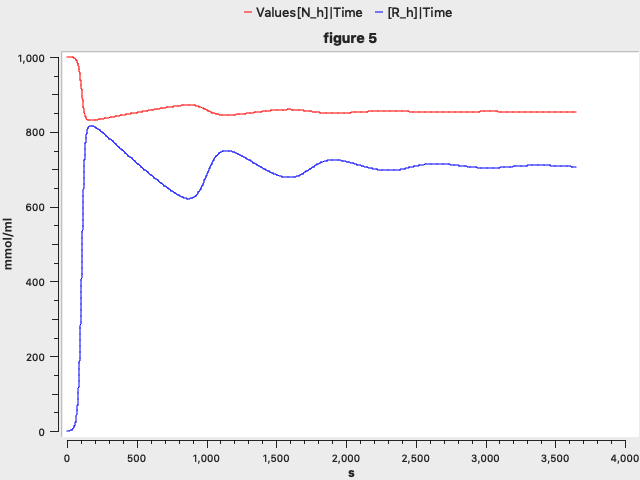## Chitnis2012 - Model Rift Valley Fever transmission between cattle and mosquitoes (Model 1)Model Identifier
BIOMD0000000950
Short description
Mathematical model for Rift Valley Fever transmission between cattle and mosquitoes without infectious eggs.
Format
SBML (L2V4)
Related Publication
• Modelling vertical transmission in vector-borne diseases with applications to Rift Valley fever.• Hyman JM, Manore CA
• Journal of biological dynamics , 1/ 2013 , Volume 7 , pages: 11-40 , PubMed ID: 23098257
• Department of Epidemiology and Public Health, Swiss Tropical and Public Health Institute, 4002 Basel, Switzerland. nakul.chitnis@unibas.ch
• We present two ordinary differential equation models for Rift Valley fever (RVF) transmission in cattle and mosquitoes. We extend existing models for vector-borne diseases to include an asymptomatic host class and vertical transmission in vectors. We define the basic reproductive number, ℛ(0), and analyse the existence and stability of equilibrium points. We compute sensitivity indices of ℛ(0) and a reactivity index (that measures epidemicity) to parameters for baseline wet and dry season values. ℛ(0) is most sensitive to the mosquito biting and death rates. The reactivity index is most sensitive to the mosquito biting rate and the infectivity of hosts to vectors. Numerical simulations show that even with low equilibrium prevalence, increases in mosquito densities through higher rainfall, in the presence of vertical transmission, can result in large epidemics. This suggests that vertical transmission is an important factor in the size and persistence of RVF epidemics.
Contributors
Matthew Roberts, Krishna Kumar Tiwari

isDescribedBy
PubMed 23098257
hasProperty
Mathematical Modelling Ontology Ordinary differential equation model
C128419
isVersionOf
occursIn
is

Curation status
Curated

Modelling approach(es)

Tags
Name Description Size Actions

### Model files

Chitnis2012_model_1.xml SBML L2V4 representation of Chitnis2012 - Model Rift Valley Fever transmission between cattle and mosquitoes (Model 1) 51.97 KB Preview | Download

Chitnis2012_model_1.cps COAPSI 4.27(217) file for the model 78.79 KB Preview | Download
• Model originally submitted by : Matthew Roberts
• Submitted: 23-May-2018 16:25:19
##### Revisions
Legends
: Variable used inside SBML models

Species
Species Initial Concentration/Amount
A h

C3833; Infection
0.0 mmol
I h

0003748; 0000460
0.0 mmol
S v

C66819; 0004757
19999.0 mmol
E v

0003748; PATO:0002425
0.0 mmol
S h

C66819; 0003748
1000.0 mmol
R h

0003748; Recovered or Resolved
0.0 mmol
I v

0000460; 0004757
1.0 mmol
Reactions
Reactions Rate Parameters
theta_h*lambda_h*S_h-(u_h+gamma_tilde_h)*A_h

theta_h*lambda_h*S_h-(u_h+gamma_tilde_h)*A_h
lambda_h = 5.143359375E-5; theta_h = 0.4; u_h = 4.5662100456621E-4; gamma_tilde_h = 0.25
(1-theta_h)*lambda_h*S_h-(u_h+gamma_h+delta_h)*I_h

(1-theta_h)*lambda_h*S_h-(u_h+gamma_h+delta_h)*I_h
delta_h = 0.1; gamma_h = 0.25; lambda_h = 5.143359375E-5; theta_h = 0.4; u_h = 4.5662100456621E-4
((N_v-psi_v*I_v)/N_v*u_v*M0-lambda_v*S_v)-u_v*S_v

((N_v-psi_v*I_v)/N_v*u_v*M0-lambda_v*S_v)-u_v*S_v
u_v = 0.05; lambda_v = 0.0; N_v = 20000.0; M0 = 20000.0; psi_v = 0.1
lambda_v*S_v-(u_v+v_v)*E_v

lambda_v*S_v-(u_v+v_v)*E_v
u_v = 0.05; lambda_v = 0.0; v_v = 0.0714285714285714
(u_h*C0-lambda_h*S_h)-u_h*S_h

(u_h*C0-lambda_h*S_h)-u_h*S_h
C0 = 1000.0; lambda_h = 5.143359375E-5; u_h = 4.5662100456621E-4
(gamma_h*I_h+gamma_tilde_h*A_h)-u_h*R_h

(gamma_h*I_h+gamma_tilde_h*A_h)-u_h*R_h
gamma_h = 0.25; u_h = 4.5662100456621E-4; gamma_tilde_h = 0.25
(psi_v*I_v/N_v*u_v*M0+v_v*E_v)-u_v*I_v

(psi_v*I_v/N_v*u_v*M0+v_v*E_v)-u_v*I_v
u_v = 0.05; N_v = 20000.0; M0 = 20000.0; v_v = 0.0714285714285714; psi_v = 0.1Curator's comment:
(added: 13 May 2020, 18:24:54, updated: 13 May 2020, 18:24:54)
Figure 5 results has been reproduced. Model encoded and plot generated using COPASI 4.27. Other figures can be generated using the setup provided in the literature.